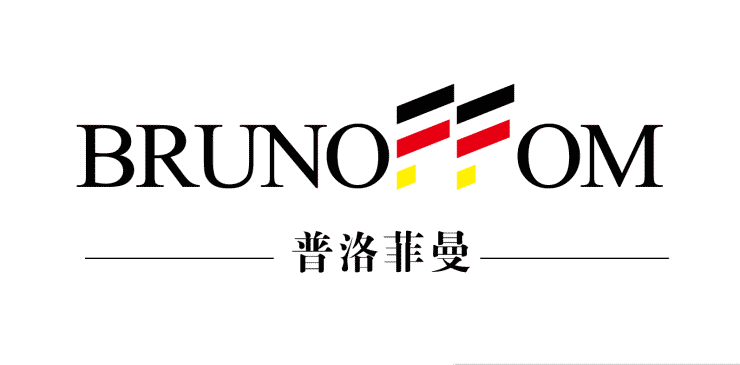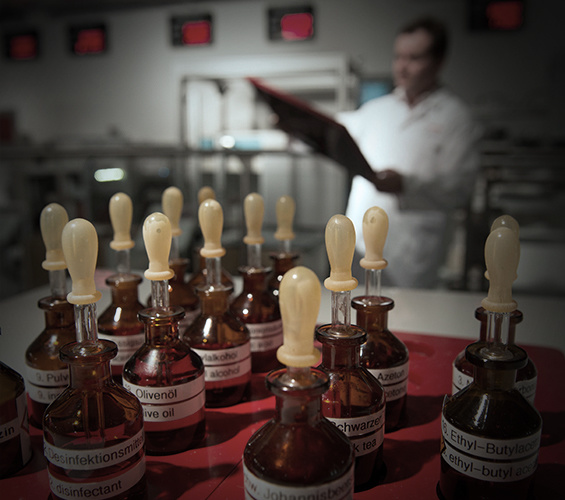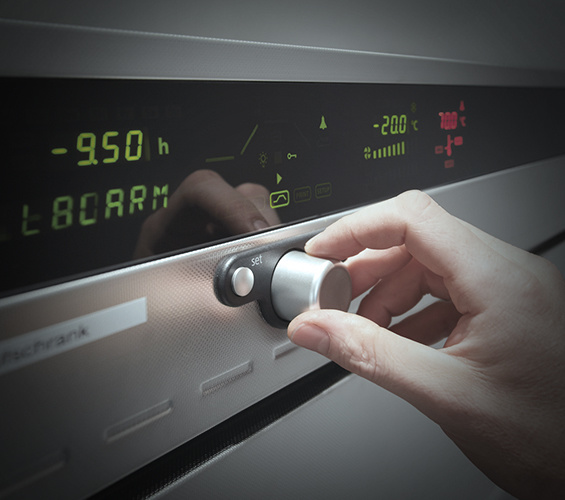|

# 普洛菲曼程强:十年砥砺沉淀,实现环保居家路

用一个十年，书写普洛菲曼在整体厨柜行业的华章；用一个十年，见证普洛菲曼董事长程强在这个行业的扎根沉淀。

普洛菲曼，一个深植环保DNA的品牌正式创立于2016年03月01日，专注环保家居十多年，荣获德国专利局颁发的厨柜、家具专用健康环保陶瓷板材、及陶瓷健康环保厨柜家具专利技术认证，是业内率先获得德国专利认证以及ISO-9001国际质量体系认证的企业，先后获得环境管理体系认证、国家十环认证品牌等国标级环保认证。“装修污染是消费者的痛点。”普洛菲曼董事长程强指出，基于这样的认识，普洛菲曼在成立之初就将品牌定位于环保、健康。而普洛菲曼董事长程强以德国精湛工艺和技术为依托，传承德国工业匠人精神和欧洲厨房设计的理念植入普洛菲曼，在生产、运营等各个层面进行系统的定位和梳理，并于2007年提出全瓷厨房的构想。

2009年成功制造出整套全瓷模型。

2010年，与蒙娜丽莎签订战略合作，开发陶瓷薄板橱柜专利，全瓷厨柜策划进入市场。

2011年，荣获德国专利局（DPMA）颁发厨柜、家具专用健康环保陶瓷板材、及陶瓷健康环保厨柜家具专利技术等两项专利认证

2012年，在普洛菲曼程强带领下，研制出第二代产品，全陶瓷厨柜准备进军市场。

2015年，第三代产品面市，持续优化整体厨房解决方案，同期注册全陶瓷厨柜生产专利3项，并广东广州第一家门店“五洲店”进入试营。

2016年，普洛菲曼品牌正式成立，并加大投资，引入德国智造理念，建立标准化自动化生产系统。携同德国唯宝陶瓷，意大利范思哲陶瓷，西班牙宝瓷兰陶瓷、西班牙德赛斯等知名供应商签订战略合作。分别在深圳、北京、武汉、东莞、长沙、贵阳、重庆、成都、上海、厦门建立子公司及专卖店。在这些环保产品陆续推出后，家居环保市场也慢慢被消费者所接受。此外，我们连续对全瓷厨房的持续推广，也让终端的经销商能更熟练地掌握。

随着消费升级浪潮的来袭，全瓷厨房的接受程度越来越高，企业纷纷打出“无醛产品”、“零醛产品”的口号，程强认为，这其实都是“伪命题”。“空气中都会有甲醛，家居产品就更不例外。”程强提到，对与家居企业来说无法做到极致的环保，只能通过板材、工艺等各个方面尽量降低甲醛含量，做到“无醛添加”，让最后呈现出来的产品甲醛含量控制在对人体接近零影响的合理范围中。在板材的运用上，普洛菲曼所采用的健康环保陶瓷板材都符合E0级环保标准；在粘合剂上，普洛菲曼优选食品级大豆蛋白胶，坚决不用会产生甲醛的脲醛树脂胶， 从源头上阻拦了甲醛在整个家具里面的释放或者伤害。“这两点其实是知易行难，毕竟作为企业，会受到商业和市场的左右，但普洛菲曼的品牌定位是环保健康，注定要站在用户的角度思考，在这方面做了很多尝试。”程强表示。他还提到，普洛菲曼从2016年开始，持续在终端店面进行产品检测活动，到后来，包括政府、地区消费者协会，以及各地的政府或民间组织，甚至是消费者都对我们产品进行抽检和测验，自发地为普洛菲曼的产品进行检测和宣传。“就这样慢慢建立起普洛菲曼的品牌口碑，这就是我们坚持环保所带来的成果。”程强表示。在绿色环保方面，普洛菲曼将把“无醛添加”的概念引进行业，让消费者对市面上所谓的”无醛产品”、“零醛产品”有正确的认知，同时，继续坚持使用健康环保陶瓷板材，这是基于企业家的良知。“在商业行为面前，企业不能失去良心，有些大规模的企业，为了避免与商业规模冲突，不会采用这种新板材，我们就慢慢地推进，希望能在社会上做出些资金的贡献。”程强说道。结语：伴随环保、健康基因而生的普洛菲曼，一直奉行和坚持打造全瓷厨房，让环保、健康的家居理念更加深入人心。这在整体厨柜行业来说，可以说是里程碑式的。我们相信，能让一个企业作为信条长期坚持且付诸实践的，除了是一个品牌定位的商业行为，更是出于一个企业的担当。

`声明：本文由入驻焦点开放平台的作者撰写，除焦点官方账号外，观点仅代表作者本人，不代表焦点立场错误信息举报电话： 400-099-0099，邮箱：jubao@vip.sohu.com，或点此进行意见反馈，或点此进行举报投诉。`A B C D E F G H J K L M N P Q R S T W X Y Z
A - B - C - D - E
• A
• 鞍山
• 安庆
• 安阳
• 安顺
• 安康
• 澳门
• B
• 北京
• 保定
• 包头
• 巴彦淖尔
• 本溪
• 蚌埠
• 亳州
• 滨州
• 北海
• 百色
• 巴中
• 毕节
• 保山
• 宝鸡
• 白银
• 巴州
• C
• 承德
• 沧州
• 长治
• 赤峰
• 朝阳
• 长春
• 常州
• 滁州
• 池州
• 长沙
• 常德
• 郴州
• 潮州
• 崇左
• 重庆
• 成都
• 楚雄
• 昌都
• 慈溪
• 常熟
• D
• 大同
• 大连
• 丹东
• 大庆
• 东营
• 德州
• 东莞
• 德阳
• 达州
• 大理
• 德宏
• 定西
• 儋州
• 东平
• E
• 鄂尔多斯
• 鄂州
• 恩施
F - G - H - I - J
• F
• 抚顺
• 阜新
• 阜阳
• 福州
• 抚州
• 佛山
• 防城港
• G
• 赣州
• 广州
• 桂林
• 贵港
• 广元
• 广安
• 贵阳
• 固原
• H
• 邯郸
• 衡水
• 呼和浩特
• 呼伦贝尔
• 葫芦岛
• 哈尔滨
• 黑河
• 淮安
• 杭州
• 湖州
• 合肥
• 淮南
• 淮北
• 黄山
• 菏泽
• 鹤壁
• 黄石
• 黄冈
• 衡阳
• 怀化
• 惠州
• 河源
• 贺州
• 河池
• 海口
• 红河
• 汉中
• 海东
• 怀来
• I
• J
• 晋中
• 锦州
• 吉林
• 鸡西
• 佳木斯
• 嘉兴
• 金华
• 景德镇
• 九江
• 吉安
• 济南
• 济宁
• 焦作
• 荆门
• 荆州
• 江门
• 揭阳
• 金昌
• 酒泉
• 嘉峪关
K - L - M - N - P
• K
• 开封
• 昆明
• 昆山
• L
• 廊坊
• 临汾
• 辽阳
• 连云港
• 丽水
• 六安
• 龙岩
• 莱芜
• 临沂
• 聊城
• 洛阳
• 漯河
• 娄底
• 柳州
• 来宾
• 泸州
• 乐山
• 六盘水
• 丽江
• 临沧
• 拉萨
• 林芝
• 兰州
• 陇南
• M
• 牡丹江
• 马鞍山
• 茂名
• 梅州
• 绵阳
• 眉山
• N
• 南京
• 南通
• 宁波
• 南平
• 宁德
• 南昌
• 南阳
• 南宁
• 内江
• 南充
• P
• 盘锦
• 莆田
• 平顶山
• 濮阳
• 攀枝花
• 普洱
• 平凉
Q - R - S - T - W
• Q
• 秦皇岛
• 齐齐哈尔
• 衢州
• 泉州
• 青岛
• 清远
• 钦州
• 黔南
• 曲靖
• 庆阳
• R
• 日照
• 日喀则
• S
• 石家庄
• 沈阳
• 双鸭山
• 绥化
• 上海
• 苏州
• 宿迁
• 绍兴
• 宿州
• 三明
• 上饶
• 三门峡
• 商丘
• 十堰
• 随州
• 邵阳
• 韶关
• 深圳
• 汕头
• 汕尾
• 三亚
• 三沙
• 遂宁
• 山南
• 商洛
• 石嘴山
• T
• 天津
• 唐山
• 太原
• 通辽
• 铁岭
• 泰州
• 台州
• 铜陵
• 泰安
• 铜仁
• 铜川
• 天水
• 天门
• W
• 乌海
• 乌兰察布
• 无锡
• 温州
• 芜湖
• 潍坊
• 威海
• 武汉
• 梧州
• 渭南
• 武威
• 吴忠
• 乌鲁木齐
X - Y - Z
• X
• 邢台
• 徐州
• 宣城
• 厦门
• 新乡
• 许昌
• 信阳
• 襄阳
• 孝感
• 咸宁
• 湘潭
• 湘西
• 西双版纳
• 西安
• 咸阳
• 西宁
• 仙桃
• 西昌
• Y
• 运城
• 营口
• 盐城
• 扬州
• 鹰潭
• 宜春
• 烟台
• 宜昌
• 岳阳
• 益阳
• 永州
• 阳江
• 云浮
• 玉林
• 宜宾
• 雅安
• 玉溪
• 延安
• 榆林
• 银川
• Z
• 张家口
• 镇江
• 舟山
• 漳州
• 淄博
• 枣庄
• 郑州
• 周口
• 驻马店
• 株洲
• 张家界
• 珠海
• 湛江
• 肇庆
• 中山
• 自贡
• 资阳
• 遵义
• 昭通
• 张掖
• 中卫

1室1厅1厨1卫1阳台

1
2
3
4
5

0
1
2

1

1

0
1
2
3报名成功，资料已提交审核A B C D E F G H J K L M N P Q R S T W X Y Z
A - B - C - D - E
• A
• 鞍山
• 安庆
• 安阳
• 安顺
• 安康
• 澳门
• B
• 北京
• 保定
• 包头
• 巴彦淖尔
• 本溪
• 蚌埠
• 亳州
• 滨州
• 北海
• 百色
• 巴中
• 毕节
• 保山
• 宝鸡
• 白银
• 巴州
• C
• 承德
• 沧州
• 长治
• 赤峰
• 朝阳
• 长春
• 常州
• 滁州
• 池州
• 长沙
• 常德
• 郴州
• 潮州
• 崇左
• 重庆
• 成都
• 楚雄
• 昌都
• 慈溪
• 常熟
• D
• 大同
• 大连
• 丹东
• 大庆
• 东营
• 德州
• 东莞
• 德阳
• 达州
• 大理
• 德宏
• 定西
• 儋州
• 东平
• E
• 鄂尔多斯
• 鄂州
• 恩施
F - G - H - I - J
• F
• 抚顺
• 阜新
• 阜阳
• 福州
• 抚州
• 佛山
• 防城港
• G
• 赣州
• 广州
• 桂林
• 贵港
• 广元
• 广安
• 贵阳
• 固原
• H
• 邯郸
• 衡水
• 呼和浩特
• 呼伦贝尔
• 葫芦岛
• 哈尔滨
• 黑河
• 淮安
• 杭州
• 湖州
• 合肥
• 淮南
• 淮北
• 黄山
• 菏泽
• 鹤壁
• 黄石
• 黄冈
• 衡阳
• 怀化
• 惠州
• 河源
• 贺州
• 河池
• 海口
• 红河
• 汉中
• 海东
• 怀来
• I
• J
• 晋中
• 锦州
• 吉林
• 鸡西
• 佳木斯
• 嘉兴
• 金华
• 景德镇
• 九江
• 吉安
• 济南
• 济宁
• 焦作
• 荆门
• 荆州
• 江门
• 揭阳
• 金昌
• 酒泉
• 嘉峪关
K - L - M - N - P
• K
• 开封
• 昆明
• 昆山
• L
• 廊坊
• 临汾
• 辽阳
• 连云港
• 丽水
• 六安
• 龙岩
• 莱芜
• 临沂
• 聊城
• 洛阳
• 漯河
• 娄底
• 柳州
• 来宾
• 泸州
• 乐山
• 六盘水
• 丽江
• 临沧
• 拉萨
• 林芝
• 兰州
• 陇南
• M
• 牡丹江
• 马鞍山
• 茂名
• 梅州
• 绵阳
• 眉山
• N
• 南京
• 南通
• 宁波
• 南平
• 宁德
• 南昌
• 南阳
• 南宁
• 内江
• 南充
• P
• 盘锦
• 莆田
• 平顶山
• 濮阳
• 攀枝花
• 普洱
• 平凉
Q - R - S - T - W
• Q
• 秦皇岛
• 齐齐哈尔
• 衢州
• 泉州
• 青岛
• 清远
• 钦州
• 黔南
• 曲靖
• 庆阳
• R
• 日照
• 日喀则
• S
• 石家庄
• 沈阳
• 双鸭山
• 绥化
• 上海
• 苏州
• 宿迁
• 绍兴
• 宿州
• 三明
• 上饶
• 三门峡
• 商丘
• 十堰
• 随州
• 邵阳
• 韶关
• 深圳
• 汕头
• 汕尾
• 三亚
• 三沙
• 遂宁
• 山南
• 商洛
• 石嘴山
• T
• 天津
• 唐山
• 太原
• 通辽
• 铁岭
• 泰州
• 台州
• 铜陵
• 泰安
• 铜仁
• 铜川
• 天水
• 天门
• W
• 乌海
• 乌兰察布
• 无锡
• 温州
• 芜湖
• 潍坊
• 威海
• 武汉
• 梧州
• 渭南
• 武威
• 吴忠
• 乌鲁木齐
X - Y - Z
• X
• 邢台
• 徐州
• 宣城
• 厦门
• 新乡
• 许昌
• 信阳
• 襄阳
• 孝感
• 咸宁
• 湘潭
• 湘西
• 西双版纳
• 西安
• 咸阳
• 西宁
• 仙桃
• 西昌
• Y
• 运城
• 营口
• 盐城
• 扬州
• 鹰潭
• 宜春
• 烟台
• 宜昌
• 岳阳
• 益阳
• 永州
• 阳江
• 云浮
• 玉林
• 宜宾
• 雅安
• 玉溪
• 延安
• 榆林
• 银川
• Z
• 张家口
• 镇江
• 舟山
• 漳州
• 淄博
• 枣庄
• 郑州
• 周口
• 驻马店
• 株洲
• 张家界
• 珠海
• 湛江
• 肇庆
• 中山
• 自贡
• 资阳
• 遵义
• 昭通
• 张掖
• 中卫• 手机• 分享
• 设计
免费设计
• 计算器
装修计算器
• 入驻
合作入驻
• 联系
联系我们
• 置顶
返回顶部